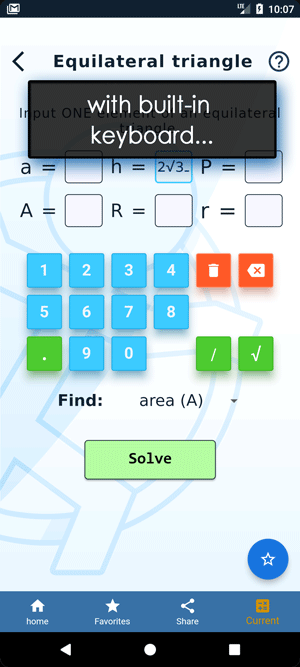Math Calculators, Lessons and Formulas

It is time to solve your math problem

mathportal.org

# 3x3 system of equations solver

This calculator solves system of three equations with three unknowns (3x3 system). The calculator will use the Gaussian elimination or Cramer's rule to generate a step by step explanation.

3x3 System of equations solver
Two solving methods + detailed steps.
show help ↓↓ examples ↓↓
 Solve by using Gaussian elimination method (default) Solve by using Cramer's rule
Find approximate solution
Hide stepsworking...
examples
example 1:ex 1:
Solve by using Gaussian elimination: \begin{aligned} x + 2y - z & = 2 \\[2ex] x - y + 2z & = 5 \\[2ex] 2x + 2y + 2z & = 12 \end{aligned}
example 2:ex 2:
Solve by using Cramer's rule \begin{aligned} -x + \frac{2}{3}y - 2z & = 2 \\[2ex] 5x + 7y - 5z & = 6 \\[2ex] \frac{1}{4}x + y - \frac{1}{2}z & = 2 \end{aligned}

This calculator uses Cramer's rule to solve systems of three equations with three unknowns. The Cramer's rule can be stated as follows:

Given the system:

\begin{aligned} a_1x + b_1y + c_1z = d_1 \\ a_2x + b_2y + c_2z = d_2 \\ a_3x + b_3y + c_3z = d_3 \end{aligned}

with

 $$D = \left|\begin{array}{ccc} a_1 & b_1 & c_1 \\ a_2 & b_2 & c_2 \\ a_3 & b_3 & c_3 \\ \end{array}\right| \ne 0$$ $$D_x = \left|\begin{array}{ccc} d_1 & b_1 & c_1 \\ d_2 & b_2 & c_2 \\ d_3 & b_3 & c_3 \\ \end{array}\right|$$ $$D_y = \left|\begin{array}{ccc} a_1 & d_1 & c_1 \\ a_2 & d_2 & c_2 \\ a_3 & d_3 & c_3 \\ \end{array}\right|$$ $$D_z = \left|\begin{array}{ccc} a_1 & b_1 & d_1 \\ a_2 & b_2 & d_2 \\ a_3 & b_3 & d_3 \\ \end{array}\right|$$

then the solution of this system is:

 $$x = \frac{D_x}{D}$$ $$y = \frac{D_y}{D}$$ $$z = \frac{D_z}{D}$$

Example: Solve the system of equations using Cramer's rule

\begin{aligned} 4x + 5y -2z= & -14 \\ 7x - ~y +2z= & 42 \\ 3x + ~y + 4z= & 28 \\ \end{aligned}

Solution: First we compute $D,~ D_x,~ D_y$ and $D_z$.

\begin{aligned} & D~~ = \left|\begin{array}{ccc} {\color{blue}{4}} & {\color{red}{~5}} & {\color{green}{-2}} \\ {\color{blue}{7}} & {\color{red}{-1}} & {\color{green}{~2}} \\ {\color{blue}{3}} & {\color{red}{~1}} & {\color{green}{~4}} \end{array}\right| = -16 + 30 - 14 - 6 - 8 - 140 = -154\\ & D_x = \left|\begin{array}{ccc} -14 & {\color{red}{~5}} & {\color{green}{-2}} \\ ~42 & {\color{red}{-1}} & {\color{green}{~2}} \\ ~28 & {\color{red}{1}} & {\color{green}{~4}} \end{array}\right| = 56 + 280 - 84 - 56 + 28 - 840 = -616\\ & D_y = \left|\begin{array}{ccc} {\color{blue}{4}} & -14 & {\color{green}{-2}} \\ {\color{blue}{7}} & ~42 & {\color{green}{~2}} \\ {\color{blue}{3}} & ~28 & {\color{green}{~4}} \end{array}\right| = 672 - 84 - 392 + 252 - 224 + 392 = 616\\ & D_Z = \left|\begin{array}{ccc} {\color{blue}{4}} & {\color{red}{~5}} & -14 \\ {\color{blue}{7}} & {\color{red}{-1}} & ~42 \\ {\color{blue}{3}} & {\color{red}{~1}} & ~28 \end{array}\right| = -112 + 630 - 98 - 42 - 168 - 980 = -770\\ \end{aligned}

Therefore,

\begin{aligned} & x = \frac{D_x}{D} = \frac{-616}{-154} = 4 \\ & y = \frac{D_y}{D} = \frac{ 616}{-154} = -4 \\ & z = \frac{D_z}{D} = \frac{-770}{-154} = 5 \end{aligned}

Note: You can check the solution using above calculator

Search our database of more than 200 calculators